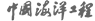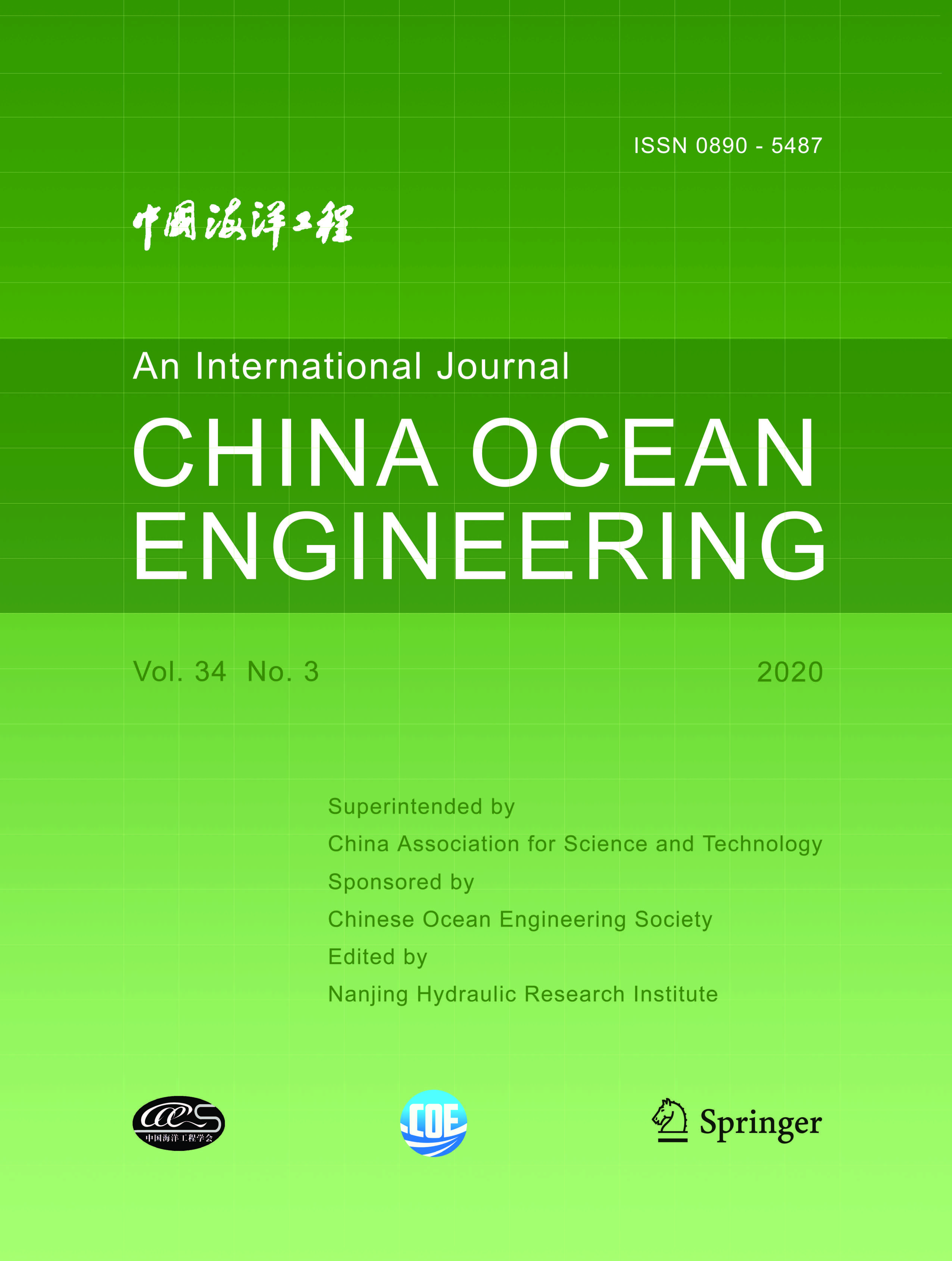ISSN  0890-5487 CN 32-1441/P

## 2000 Vol.(2)

Display Mode：          |

2000, (2): -.
[Abstract](347)
Abstract:
An efficient numerical model for wave refraction, diffraction and reflection is presented in this paper. In the model, the modified time-dependent mild-slope equation is transformed into an evolution equation and an improved ADI method involving a relaxation factor is adopted to solve it. The method has the advantage of improving the numerical stability and convergence rate by properly determining the relaxation factor. The range of the relaxation factor making the differential scheme unconditionally stable is determined by stability analysis. Several verifications are performed to examine the accuracy of the present model. The numerical results coincide with the analytic solutions or experimental data very well, and the computer time is reduced.
2000, (2): -.
[Abstract](357)
Abstract:
The river reach downstream of a floodgate at the estuary of the Xinyihe River is about 1.3km long, and the riverbed is composed of clotty clay. In the experiment, soil samples are taken from the construction site, and the incipient velocity is determined in a laboratory flume, and it is used to design the scour model and to select model sand material. The experimental results show that scours below the floodgate is unavoidable due to large discharge and low tidal level. Scours is caused by two factors: the rapid flow passing though the floodgate and the water drop near the river mouth during low tide, and the scour below the floodgate is more critical to the structural design. It is suggested that anti-scour walls should be used instead of riprap. The ideas and methods adopted in the experiment can be used as reference in the study on river scour under similar conditions.
2000, (2): -.
[Abstract](343)
Abstract:
This paper deals with several hot topics in ice related problems. In recent years, advances have been made on ice breaking modes, dynamic ice loads on offshore structures, ice-induced structural vibrations, fatigue and fracture by ice-structure interaction, and design of jackets in the Bohai Gulf.
2000, (2): -.
[Abstract](330)
Abstract:
Studies on the possible effects of a detached breakwater on the characteristics of the wave field are carried out experimentally. A serpentine wave generator is used to generate both uni- and multi- directional waves. Characteristics of the wave fields analyzed here include the wave field directionality, and the probability distributions of surface elevations and of the wave heights. Owing to the presence of the breakwater, waves outside the harbour are found to be reflected with, however, concentrated energy within the harbour entrance. In general, wave heights can be approximated with a Rayleigh distribution, with occasional deviations from the theory. This occurs more frequently for waves with higher peak frequency values than for those with lower values both for uni- and multi-directional waves. Surface elevations can be approximated with the Gaussian model, although the Edgeworth's form of the type A Gram- Charlier series expansions would yield better fits. Wave directionality is found to have no discernible effects on the statistical characteristics of the wave field.
2000, (2): -.
[Abstract](322)
Abstract:
The dynamic response of offshore platforms is more serious in hostile sea environment than in shallow sea. In this paper, a hybrid solution combined with analytical and numerical method is proposed to compute the stochastic response of fixed offshore platforms to random waves, considering wave-structure interaction and non-linear drag force. The simulation program includes two steps: the first step is the eigenanalysis aspects associated the structure and the second step is response estimation based on spectral equations. The eigenanalysis could be done through conventional finite element method conveniently and its natural frequency and mode shapes obtained. In the second part of the process, the solution of the offshore structural response is obtained by iteration of a series of coupled spectral equations. Considering the third-order term in the drag force, the evaluation of the three-fold convolution should be demanded for nonlinear stochastic response analysis. To demonstrate this method, a numerical analysis is carried out for both linear and non-linear platform motions. The final response spectra have the typical two peaks in agreement with reality, indicating that the hybrid method is effective and can be applied to offshore engineering.
2000, (2): -.
[Abstract](323)
Abstract:
A numerical study is presented on roll damping of ships by solving Navier-Stokes equation. Two-Dimensional unsteady incompressible viscous flow around the rolling cylinders of various ship-like cross sections are numerically simulated by use of the computational scheme previously developed by the authors. The numerical results show that the location of the vortices is very similar to the existing experimental result. For comparison of vortex patterns and roll damping on various ship-like cross sections, various distributions of shear stress and pressure on the rolling ship hull surface are presented in this paper. It is found that there are two vortices around the midship-like section and there is one vortex around the fore or stern section. Based on these simulation results, the roll damping of a ship including viscous effects is calculated. The contribution of pressure to the roll moment is larger than the contribution of frictional shear stress.
2000, (2): -.
[Abstract](352)
Abstract:
For measurement of sediment spatial concentration of nearshore sea water, a turbid water system with several kinds of particles is investigated from the viewpoint of the characteristics of particles. Firstly, the classical physical and chemical conductivity formula is extended to macro-particle (such as sand) conductivity formula. Secondly, the Fricke formula suitable for only one kind of particles is extended to the conductivity formula suitable for several kinds of particles. Finally, the multi-particle conductivity formula is applied to the measurement of sediment spatial concentration.
2000, (2): -.
[Abstract](334)
Abstract:
It is well known that energy spectrum bandwidth should be able to reflect the degree of energy concentration. However, the commonly used bandwidth factors defined by Longuet-Higgins could.not fit the concept satisfactorily. A new kind of spectrum bandwidth scale factor with a clear physical meaning is given in the present paper and a constant is obtained which reveals the intrinsic characteristics of sea waves. Thereby a universal relationship between significant wave height of sea waves and spectrum bandwidth is established.
2000, (2): -.
[Abstract](324)
Abstract:
By means of a logarithm law for the velocity profile, a corrected formula of bed resistance coefficient, which involves many factors such as gradient of still water depth, variation of surface elevation, flow direction, and so on, is derived from the 3D governing equations of tidal current by averaging over the whole water depth. Theoretical analysis and application have shown that the 2D plane tidal current numerical model would be more reasonable and could be applied to steep bottom topography when the corrected bed resistance coefficient is used, therefore the results of reproduction simulation and engineering calculation would be more scientific and reasonable.
2000, (2): -.
[Abstract](339)
Abstract:
2000, (2): -.
[Abstract](330)
Abstract:
The history of forecasting wind waves by wave energy conservation equation is briefly described. Several currently used wave numerical models for shallow water based on different wave theories are discussed. Wave energy conservation models for the simulation of shallow water waves are introduced, with emphasis placed on the SWAN model, which takes use of the most advanced wave research achievements and has been applied to several theoretical and field conditions. The characteristics and applicability of the model, the finite difference numerical scheme of the action balance equation and its source terms computing methods are described in detail. The model has been verified with the propagation refraction numerical experiments for waves propagating in following and opposing currents; finally, the model is applied to the Haian Gulf area to simulate the wave height and wave period field there, and the results are compared with observed data.
2000, (2): -.
[Abstract](340)
Abstract:
The lateral vibration differential equation for a marine riser conveying fluid is derived by use of the small deflection theory, and the effect of internal flow velocity and top tension on the natural frequency of the riser is studied by use of FEM. At the same time, the preliminary relationship between the natural frequency and riser span under different internal flow velocities is obtained, the effect of riser supports on the vibration frequency is computed. It is found that the natural frequency of the marine riser increases with the increase of top tension, however decreases with the increase of internal flow velocity. In addition, the frequency decreases drastically with the increase of riser span.
2000, (2): -.
[Abstract](319)
Abstract:
The elliptic mild slope equation is used to simulate linear wave propagation over variable sea bed topography with mild slopes. The governing equation is discretized by the finite difference method. Based on the BI-CGSTAB technique, an attractive variant of Bl-Conjugate Gradients (BI-CG) method, the obtained linear algebraic system of equations is solved. Numerical experiments show that the BI-CGSTAB method is efficient for solving the elliptic mild slope equation. The results obtained by the BI-CGSTAB-Ba-sed method are much the same as those obtained by other authors with different solution methods, but the convergence rate is much faster than that of other methods.

### Current Issue1. Editor-in-Chief:

2. Superintended by:

CHINA ASSOCIATION FOR SCIENCE AND TECHNOLOGY

Chinese Ocean Engineering Society （COES）

4. Edited by:

Nanjing Hydraulic Research InstituteAddress: 34 Hujuguan, Nanjing 210024, China Pos: 210024 Tel: 025-85829388 E-mail: coe@nhri.cn

Support by Beijing Renhe Information Technology Co. Ltd E-mail: info@rhhz.net